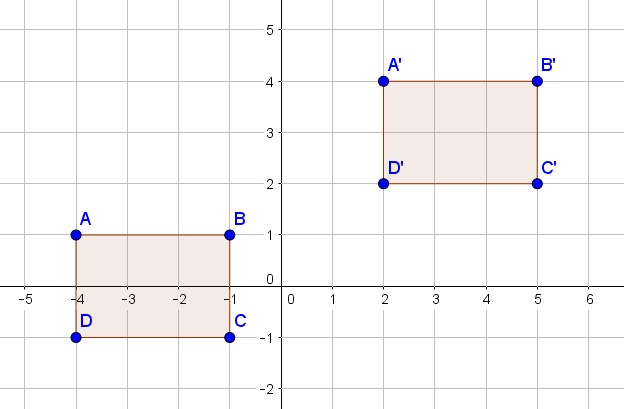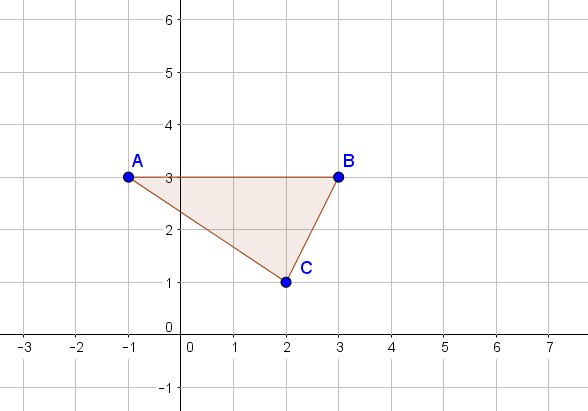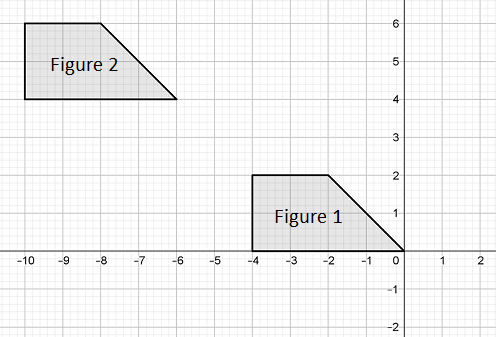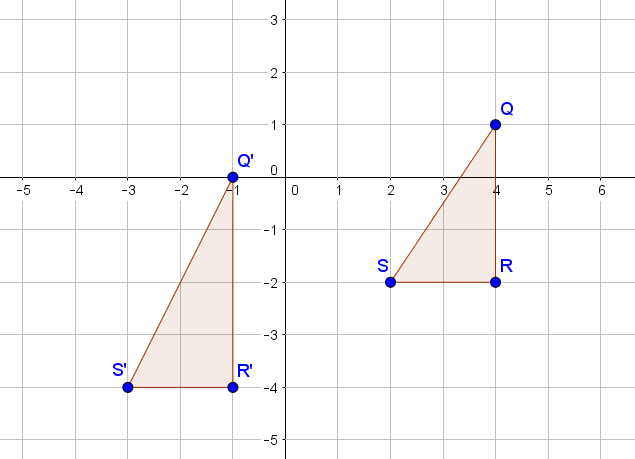Match Fishtank is now Fishtank Learning!

# Transformations and Angle Relationships

## Objective

Describe and perform translations between congruent figures. Use translations to determine if figures are congruent.

## Common Core Standards

### Core Standards

?

• 8.G.A.1.A — Lines are taken to lines, and line segments to line segments of the same length.

• 8.G.A.1.B — Angles are taken to angles of the same measure.

• 8.G.A.1.C — Parallel lines are taken to parallel lines.

• 8.G.A.2 — Understand that a two-dimensional figure is congruent to another if the second can be obtained from the first by a sequence of rotations, reflections, and translations; given two congruent figures, describe a sequence that exhibits the congruence between them.

## Criteria for Success

?

1. Describe a translation between two congruent figures using direction and magnitude.
2. Understand that when a translation is performed on a figure, all of the points on the figure move the exact same distance and in the same direction.
3. Understand that a translation preserves orientation, shape, and size of a figure but not location.

## Tips for Teachers

?

• Lessons 2 and 3 are on translations. Lesson 2 focuses on students understanding how to perform translations and how to talk about them. Students should now be starting to use the mathematical language "translation", compared to more informal vocabulary used in Lesson 1.
• The following materials are useful for this lesson: patty paper (or transparency paper), graph paper.

#### Remote Learning Guidance

If you need to adapt or shorten this lesson for remote learning, we suggest prioritizing Anchor Problem 1 (benefits from discussion) and Anchor Problem 2 (benefits from worked example). Find more guidance on adapting our math curriculum for remote learning here.

#### Fishtank Plus

• Problem Set
• Student Handout Editor
• Vocabulary Package

## Anchor Problems

?

### Problem 1

You want to prove that ${{ABCD}}$ and ${{A'B'C'D'}}$ are congruent by using a translation. Explain how you could translate ${{ABCD}}$ onto ${{A'B'C'D'}}$ so that they overlap perfectly. Be specific and use the coordinate plane as a reference.### Problem 2

Translate Figure ${ABC}$ 3 units to the right and 2 units up. Name and label the new figure.1. How are the two figures the same?
2. How are the two figures different?

### Problem 3

FIgure 1 is congruent to Figure 2.Which statement demonstrates the congruency between the two figures?

1. Figure 1 is translated 2 units left and 2 units up to Figure 2.
2. Figure 1 is translated 2 units right and 2 units down to Figure 2.
3. Figure 1 is translated 6 units left and 4 units up to Figure 2.
4. Figure 1 is translated 6 units right and 4 units down to Figure 2.

## Problem Set

?The following resources include problems and activities aligned to the objective of the lesson that can be used to create your own problem set.

• Examples where students perform translations and explain how/why the figures are congruent, similar to Anchor Problem #2
• Examples where students describe translations between two congruent figures, similar to Anchor Problems #1 and #3
• Examples where students determine if two figures are congruent; be sure to include examples where the figures are not congruent due to a change in the shape
• Example where two students debate the order in which a translation was performed; explain why both students could be correct
• GeoGebra can be used to create translated figures in the coordinate plane.

?

Two figures are shown in the coordinate plane. Alex thinks that the two figures are congruent because Figure ${QRS}$ could be translated 5 units to the left and 2 units down to map to Figure ${Q'R'S'}$1. Explain why Alex’s thinking is incorrect.
2. How would you change Figure ${Q'R'S'}$ so it is congruent to Figure ${QRS}$? What translation would prove the congruence between the two figures?

?